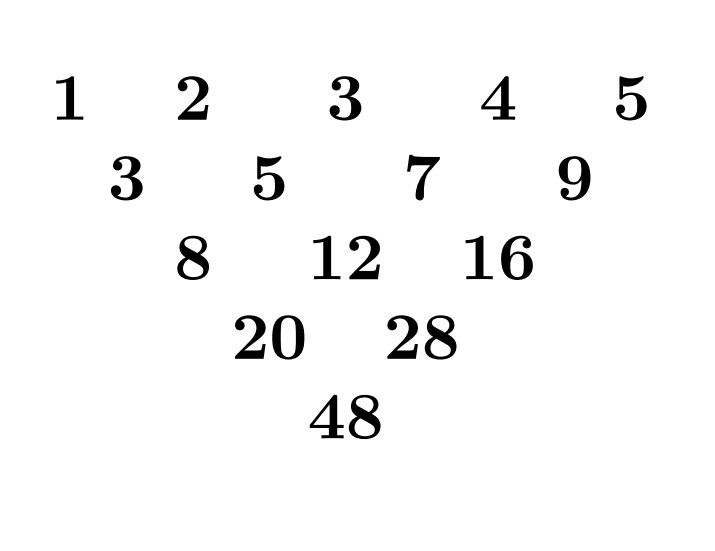# Let's call this as the Mohanty's Triangle!

Logic Level 4A triangle like the one shown above is constructed with the numbers from 1 to 2015 in the first row. Each number in the triangle, except those in the first row, is the sum of the two numbers above it. Let $N$ be the number that occupies the lowest vertex of the triangle.

If the prime factorization of $N$ is $p_1^{k_1} \cdot p_2^{k_2} \cdot p_3^{k_3},$ find the value of $p_1 + p_2 + p_3 + k_1 + k_2 + k_3$.

×